# Scale factor 2

Nadeem has a 10 inch by 10 inch photo album. He wants to make as large a copy of the original photo as possible to fit the album. Whats are the largest dimensions the copy can be if he keeps the proportion of the original photo? Explain your answer. .. . original photo is 5 inches by 8 inches.

x =  6.25 inch
y =  10 inch

### Step-by-step explanation: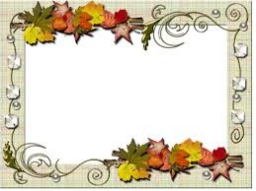Did you find an error or inaccuracy? Feel free to write us. Thank you!Tips to related online calculators
Do you want to convert length units?

#### You need to know the following knowledge to solve this word math problem:

We encourage you to watch this tutorial video on this math problem:

## Related math problems and questions:

• Photo enlarge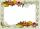Mark wants to enlarge a 4-inch by 6-inch photo so that it has a height of 15inches. Use a ratio table to determine the new width of the photo.
• A map 4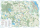A map represents every 4 miles with 1 inch. If a school and a bank are actually 12 miles apart, how far apart are they on the map, in inches?
• Map 2At what scale is made map if the distance 8.2 km corresponds on the map segment 5 cm long?
• Map scale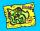What is the scale of the map, if 5.4 km is 72 mm on the map?
• Two villages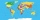Two villages are 11 km and 500 m away. On the map, their distance is determined by a 5 cm long line. Find the scale of the map.
• Map scaleGarden has on plan on a scale of 1: 150 width 22 cm and length 35 cm. What is the real area of the garden?
• The scaleThe distance of 15 cm on the map corresponds to a distance of 27 km in reality. What is the scale of the map?
• Map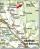Forest has an area of ​​36 ha. How much area is occupied by forest on the map at scale 1:500?
• Two citiesOn a map, 1.5 cm represents 50 miles. If the distance between the two cities on the map is 6 cm, how many miles separate the cities?
• A sculptor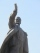A sculptor is duplicating a statue based on an original statue. If the scale factor of the replication is 3.2, will the new statue be larger or smaller than the original statue (enter 1 = larger, 0= smaller)
• PlotThe plot on which Mr. Kalous is to build a house has the shape of a rectangle. On a 1: 500 scale, its dimensions are 7cm and 5.5cm. Find out the dimensions of the plot. Calculate the parcel size.
• Plan of the villagePlan of the municipality in 1:1000 scale has plotted garden with dimensions 25 mm and 28 mm. Determine the area of gardens in ares in reality.
• Scale of the mapDetermine the scale of the map if the actual distance between A and B is 720 km and distance on the map is 20 cm.
• A map 3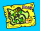A map is drawn to a scale of 1 : 25 000. This scale can be expressed as 1 cm represents n km. Find n.
• Map scaleThe rectangular plot has a scale of 1: 10000 area 3 cm2 on the map. What content does this plot have on a 1:5000 scale map?
• Scale of the map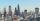The distance between two cities is actually 30 km and the map is 6 cm. What is the scale of the map?
• On the mapA line 1.5 cm long corresponds to a line 3 cm long on the map. What is the scale of the map?